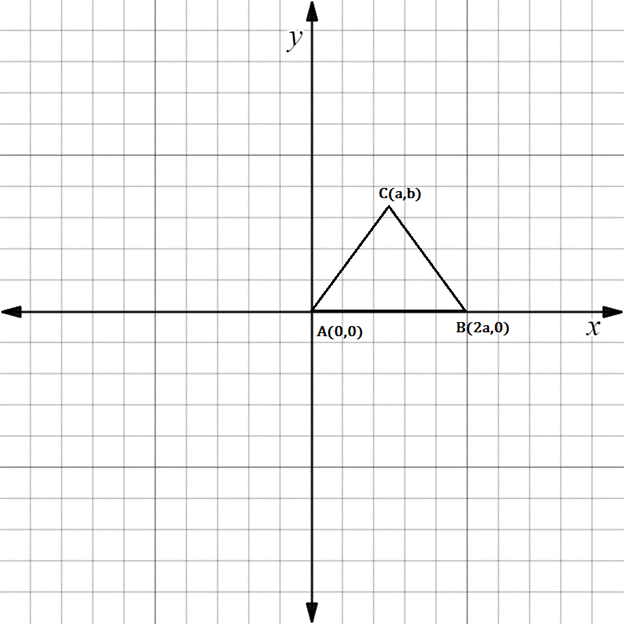Chapter 10.3, Problem 27EElementary Geometry For College St...

7th Edition
Alexander + 2 others
ISBN: 9781337614085

Solutions

Chapter
SectionElementary Geometry For College St...

7th Edition
Alexander + 2 others
ISBN: 9781337614085
Textbook Problem

In Exercises 23 to 28, find the equation (realtionship) requested. Then eliminate fractions and square root radicals from the equation.Suppose that △ A B C is an equilateral triangle. State an equation that relates variables a and b .To determine

To state:

An equation that relates a and b for the given figure.

Explanation

The given figure is shown below.

For an equilateral triangle, all sides are equal in length.

AB=BC=AC

Let us take any two sides and equate them.

AB=AC

To find AB, we have to use the distance formula.

x1,y1=A0, 0

x2,y2=B2a, 0

Formula for distance between two points

d=x2-x12+y2-y12

Substituting the x and y co-ordinates

dAB=2a-02+0-02

On solving this,

dAB=2a2+02

dAB=4a2

To find AC, we have to use the distance formula

Still sussing out bartleby?

Check out a sample textbook solution.

See a sample solution

The Solution to Your Study Problems

Bartleby provides explanations to thousands of textbook problems written by our experts, many with advanced degrees!

Get Started

Convert the expressions in Exercises 6584 to power form. 25x3

Finite Mathematics and Applied Calculus (MindTap Course List)

Sketch the region in the xy-plane. 47. {(x, y) | |x| 2}

Single Variable Calculus: Early Transcendentals, Volume I

Find the derivatives of the functions in Problems 1-34. 33.

Mathematical Applications for the Management, Life, and Social Sciences

Differentiate. y=tsint1+t

Single Variable Calculus: Early Transcendentals